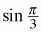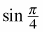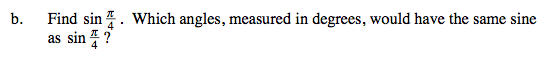### Home > A2C > Chapter 8 > Lesson 8.1.5 > Problem8-78

8-78.
1. Your scientific or graphing calculator can function in both degrees and radians. See if you can figure out how to put your calculator in radian mode and then how to switch it back to degree mode. On most scientific calculators, a small “DEG” or “RAD” shows on the screen to let you know which mode you are in. Homework Help ✎

1. With your calculator in degree mode, find sin 60° and record your answer. Then switch to radian mode and find. Did you get the same answer? Explain why your answers should be the same or different.

2. Find. Which angles, measured in degrees, would have the same sine as?$\text{What is }60\degree\text{ in radians? } \frac{\pi}{3}\text{ in degrees?}$45°, 135°', 405°, etc.